#There are 366 different Starters of The Day, many to choose from. You will find below some starters on the topic of Circles. A lesson starter does not have to be on the same topic as the main part of the lesson or the topic of the previous lesson. It is often very useful to revise or explore other concepts by using a starter based on a totally different area of Mathematics.

Main Page

### Circles Starters: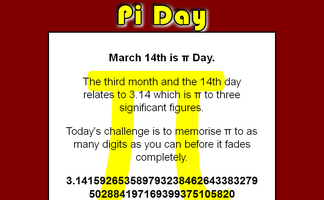March 14th is Pi Day. The third month and the 14th day relates to 3.14 which is pi to three significant figures. How many figures of pi can you memorise?It is called Refreshing Revision because every time you refresh the page you get different revision questions.Write down the names of all the mathematical shapes you know.

## Exercises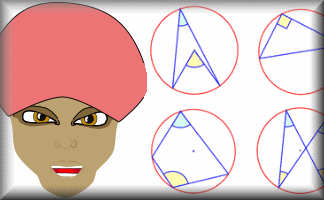#### Angle Theorem Kim's Game

A memory game to be projected to help the whole class revise the circle angle theorems.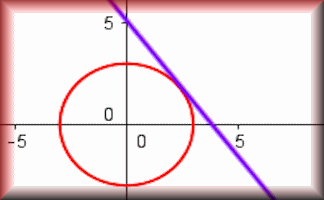#### Circle Equations

Recognise and use the equation of a circle with centre at the origin and the equation of a tangent to a circle.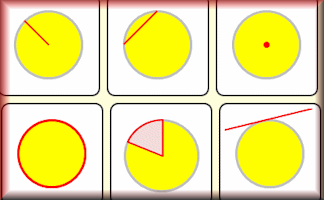#### Circle Pairs

Find the matching pairs of circle diagrams and circle properties in this interactive online game.#### Circle Parts Kim's Game

A memory game to be projected to help the whole class revise the names for the parts of a circle.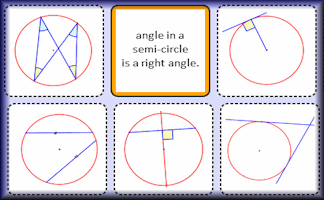#### Circle Theorem Pairs

A pairs game based around ten theorems about the angles made with chords, radii and tangents of circles.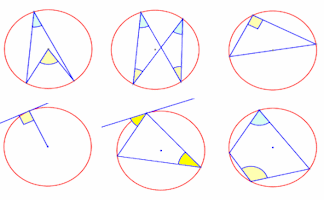#### Circle Theorems

Diagrams of the circle theorems to be projected onto a white board as an effective visual aid.#### Circle Theorems Exercise

Show that you understand and can apply the circle theorems with this self marking exercise.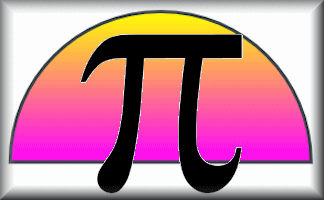#### Circles

Practise using pi to calculate various circle measurements. There are six levels of difficulty.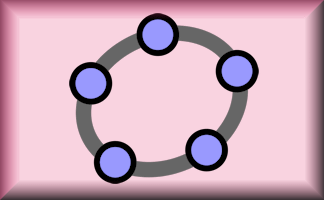#### Geometry Toolbox

Create your own dynamic geometrical diagrams using this truly amazing tool from GeoGebra.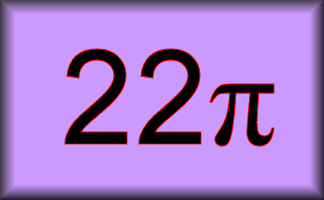#### In Terms of Pi

Circle related questions requiring the answer to include a multiple of pi rather than an approximation.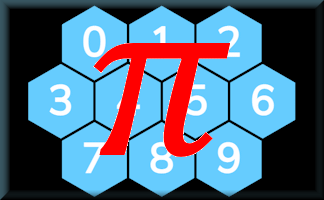#### Pi-Mon

Memorise the digits of pi with help from some musical notes.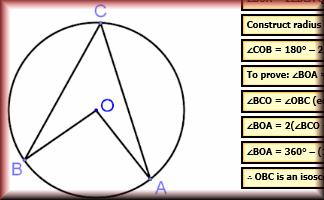#### Proof of Circle Theorems

Arrange the stages of the proofs for the standard circle theorems in the correct order.

### Search

The activity you are looking for may have been classified in a different way from the way you were expecting. You can search the whole of Transum Maths by using the box below.

Have today's Starter of the Day as your default homepage. Copy the URL below then select
Tools > Internet Options (Internet Explorer) then paste the URL into the homepage field.

Set as your homepage (if you are using Internet Explorer)

Do you have any comments? It is always useful to receive feedback and helps make this free resource even more useful for those learning Mathematics anywhere in the world. Click here to enter your comments.For All: